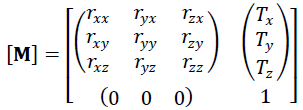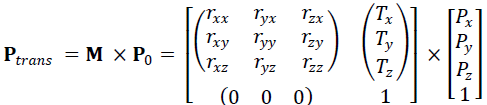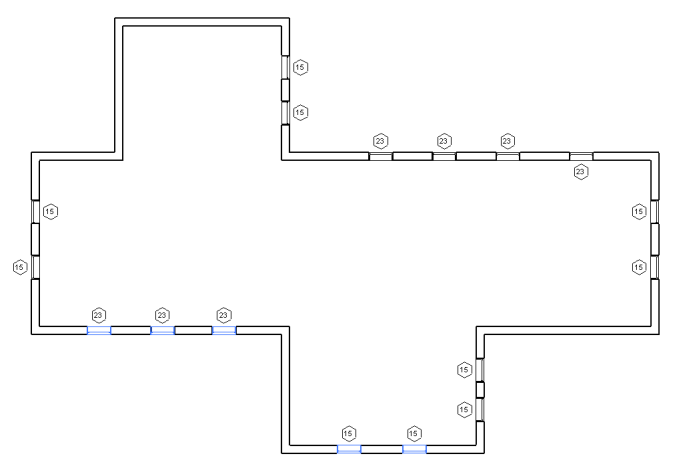### Transformations

This is part 7 of Scott Conover's AU 2009 class on analysing building geometry, exploring coordinate transformations and the placement and orientation of family instances. For completeness sake, here are some back pointers to previous blog posts dealing with transforms and related issues:

So now I hand the word back to Scott:

#### Coordinate Transformations

Revit and the Revit API use coordinate transformations in a variety of ways. In most cases, transformations are represented in the API as Transform objects. A transform object represents a homogenous transformation combining elements of rotation, translation, and less commonly, scaling and reflection:In this matrix, the 3 x 3 matrix represents the rotation applied via the transform, while the 1 x 3 matrix represents the translation. Scaling and mirror properties, if applied, show up as multipliers on the matrix members. Mathematically, a transformation of a point looks like this:In the Transform class you have direct access to the members of the transformation matrix:

• The properties BasisX, BasisY, and BasisZ are the unit vectors of the rotated coordinate system.
• The Origin property is the translation vector.

Use the Transform.OfPoint() and Transform.OfVector() methods to apply the transformation to a given input point or vector. You can also use the Transformed indexed properties (on Curve, Profile, and Mesh) to transform geometry you extracted from Revit into an alternate coordinate system.

You obtain a transform from a variety of operations:

• From the Instance class: the transformation applied to the instance.
• From a Reference containing an Instance.
• From a Panel (curtain panel) element.
• From a 3D bounding box.
• From static properties on Transform: .Identity, .Reflection, .Rotation, .Translation.
• By multiplying two Transforms together: Transform.Multiply().
• By scaling a transform and possibly its origin: Transform.ScaleBasis() and Transform.ScaleBasisAndOrigin().
• By constructing one from scratch: Transform constructor.

Typically Transforms are right-handed coordinate systems, but mirror operations will produce left-handed coordinate systems. Note that Revit sometimes produces left-handed coordinate systems via flip operations on certain elements.

#### Transformation of Instances

The Instance class stores geometry data in the SymbolGeometry property using a local coordinate system. It also provides a Transform instance to convert the local coordinate system to a world coordinate space. To get the same geometry data as the Revit application from the Instance class, use the transform property to convert each geometry object retrieved from the SymbolGeometry property.

#### South facing windows

Similarly to the example selecting all south facing walls, we can use the transform of the Instance stored in a window FamilyInstance to find south facing windows:The Y direction of the transform is the facing direction; however, the flipped status of the window affects the result. If a family instance is flipped in X, its FlippedHand property is true. If it is flipped in Y, its FlippedFacing property is true. If either one of these is set, and the other not, the result must be reversed. Here is the GetWindowDirection method implementing this:

```protected XYZ GetWindowDirection( FamilyInstance window )
{
Options options = new Options();

// Extract the geometry of the window.

Autodesk.Revit.Geometry.Element geomElem
= window.get_Geometry( options );

foreach( GeometryObject geomObj in geomElem.Objects )
{
// We expect there to be one main Instance
// in each window.  Ignore the rest of the geometry.

Instance instance = geomObj as Instance;
if( instance != null )
{
// Obtain the Instance transform and the
// nominal facing direction (Y-direction).

Transform t = instance.Transform;

XYZ facingDirection = t.BasisY;

// If the window is flipped in one direction,
// but not the other, the transform is left handed.
// The Y direction needs to be reversed to
// obtain the facing direction.

if( ( window.FacingFlipped && !window.HandFlipped )
|| ( !window.FacingFlipped && window.HandFlipped ) )
{
facingDirection = -facingDirection;
}

// Because the need to perform this operation
// on instances is so common, the Revit API exposes
// this calculation directly as the FacingOrientation
// property as shown in GetWindowDirectionAlternate()

return facingDirection;
}
}
return XYZ.BasisZ;
}
```

Because the need to perform this operation on instances is so common, the Revit API exposes this calculation directly as the FacingOrientation property:

```protected XYZ GetWindowDirectionAlternate(
FamilyInstance window )
{
return window.FacingOrientation;
}
```

#### Nested instances

Note that instances may be nested inside other instances. Each instance has a Transform which represents the transformation from the coordinates of the symbol geometry to the coordinates of the instance. In order to get the coordinates of the geometry of one of these nested instances in the coordinates of the document, you will need to concatenate the transforms together using Transform.Multiply().

Next, we look at the Revit project location, which also affects the location and transformations of the elements in the project.